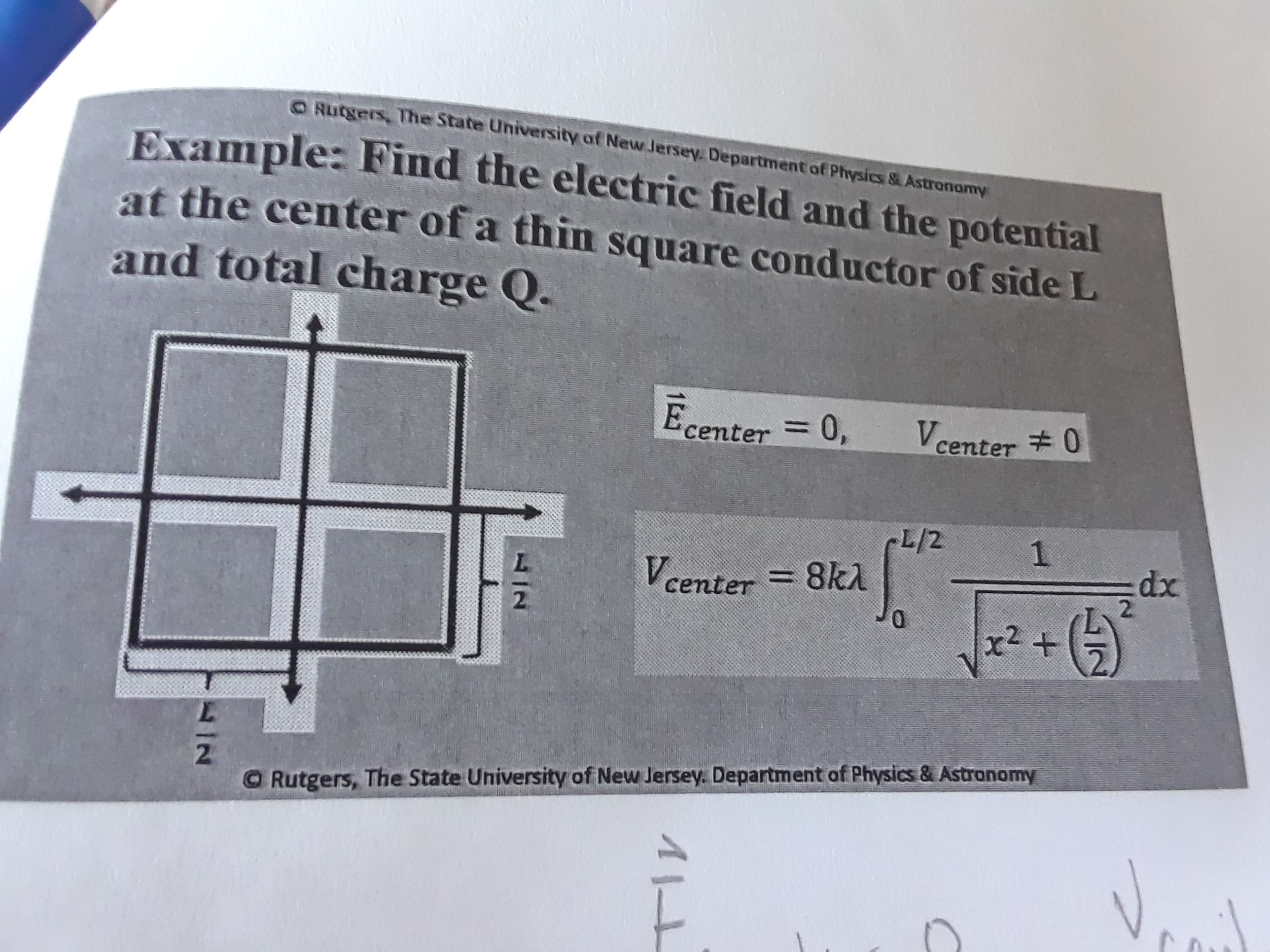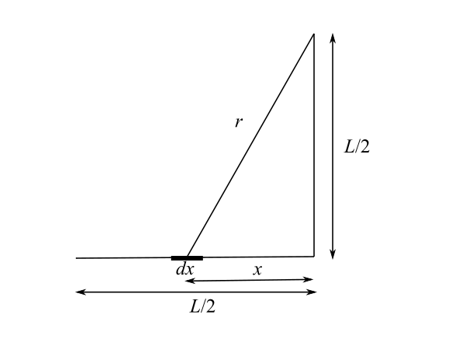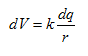# O Rutgers, The State University of New Jersey. Department of Physics&AstronormyExample: Find the electric field and the potentialat the center of a thin square conductor of side Land total charge Q.Ecenter 0,Vcenter 0L/28k11xp:dx2.center=2+r22O Rutgers, The State University of New Jersey. Department of Physics & Astranomy

Question
2 views

I do not understand how the professor came to the conclusion for the Vcenter equation.help_outlineImage TranscriptioncloseO Rutgers, The State University of New Jersey. Department of Physics&Astronormy Example: Find the electric field and the potential at the center of a thin square conductor of side L and total charge Q. Ecenter 0, Vcenter 0 L/2 8k1 1 xp :dx 2. center= 2 + r2 2 O Rutgers, The State University of New Jersey. Department of Physics & Astranomy fullscreen
check_circle

Step 1

The potential at the center of the square would be given by the sum of individual potentials at the center due to conductor segments of length L/2. Consider the diagram below of one such segment.

Step 2Step 3

The potential due an length element dx of the conductor at the center is given as,Here, dq is the charge in dx length, k is the Coulomb’s constant and r is the line element’s distance from the center.

Integrate above equation to obtain the potential at the cen...

### Want to see the full answer?

See Solution

#### Want to see this answer and more?

Solutions are written by subject experts who are available 24/7. Questions are typically answered within 1 hour.*

See Solution
*Response times may vary by subject and question.
Tagged in

### Physics